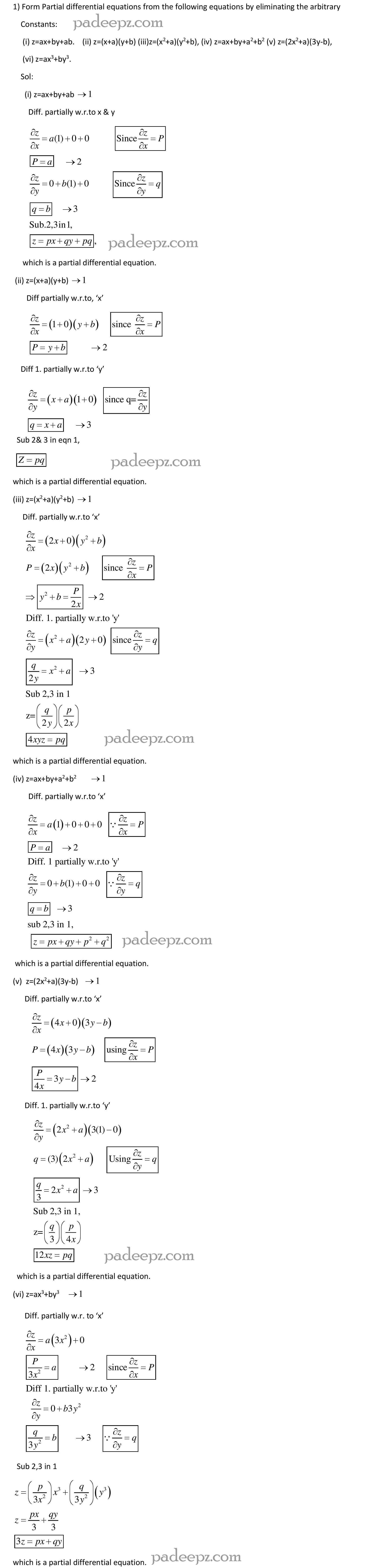Categories

# MA8353 Question Paper Transforms and Partial Differential Equations

MA8353 Question Paper Transforms and Partial Differential Equations Regulation 2017 Anna University free download. Transforms and Partial Differential Equations Question Paper MA8353 pdf free download.

## Sample MA8353 Question Paper Transforms and Partial Differential Equations:

1. What is the basic difference between the solution of one dimensional wave
equation and one dimensional heat equation?

2. In steady state conditions derive the solution of one dimensional heat flow
equation.

3. What are the possible solutions of one dimensional wave equation? MA8353 Question Paper Transforms and Partial Differential Equations

4. State one dimensional heat equation with the initial and boundary conditions.

5. Write the boundary conditions and initial conditions for solving the vibration of
string equation, if the string is subjected to initial displacement f (x) and initial
velocity g(x).

6. Write down the governing equation of two dimensional steady state heat
conduction.

7. Write down three possible solutions of one dimensional and two dimensional
steady state heat flow equation. MA8353 Question Paper Transforms and Partial Differential Equations

8. A rod 40 cm long with insulated sides has its ends A and B kept
at c  20 and c  60 respectively. Find the steady state temperature at a location 15 cm from A.

9. Form the partial differential equation by eliminating a and b from z  a(x  y)  b MA8353 Question Paper Transforms and Partial Differential Equations

10. Form the partial differential equation by eliminating a and b from ( )( ) 2 2 2 2 z  x  a y  b

11. Form the partial differential equation by eliminating from z  a x  ay  b 2 2

12. Form the partial differential equation by eliminating a and b from 2 2 z  (x  a)  (y  b)

13. Find the singular integral of the PDE 2 2 z  px  qy  p  q

14. Solve (3z  4y)p  (4x  2z)q  (2y  3x)

15. Solve ( ) ( ) ( ) 2 2 2 2 x y  z p  y x  z q  z x  y

16. Solve ( ) ( ) ( ) 2 2 2 2 2 2 x z  y p  y x z q  z y  x

17. Solve x  yz p  y  xz q  z  xy 2 2 2 ( ) ( )

18 .Solve (D 3DD’ 2D’ )z sin x cos y MA8353 Question Paper Transforms and Partial Differential Equations

 Subject name Transforms and Partial Differential Equations Semester 3 Subject Code MA8353 Regulation 2017 regulation

MA8353 Question Bank Transforms and Partial Differential Equations

MA8353 Important questions Transforms and Partial Differential Equations

MA8353 Syllabus Transforms and Partial Differential Equations

MA8353 Notes Transforms and Partial Differential Equations

### Padeepz E-Learning Materials MA8353 Transforms and Partial Differential Equations

Padeepz E-Learning Materials for MA8353 Transforms and Partial Differential Equations we have provided the sample materials in this page.  If you like the sample and want to buy the full subject the procedure is also provided in this page.Hi Padeepz.com is a place where we make Engineering Students to understand their subjects in an easy and in a effective way. Which leads to greater knowledge in the subject and Provide plenty of time spending in innovative ideas.